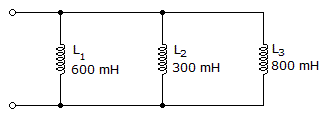# Electronics - Inductors - Discussion

### Discussion :: Inductors - General Questions (Q.No.10)

10.What is the total inductance in the given circuit?

 [A]. 160 mH [B]. 300 mH [C]. 900 mH [D]. 1700 mH

Explanation:

No answer description available for this question.

 Gjhoj said: (Nov 9, 2011) 1/600+1/300+1/800 .006+.003+.008

 Tejas Savani said: (Apr 6, 2012) Total= 1/[(1/L1) + (1/L2) + (1/L3)] = 1/0.00625 = 160 mH

 Diwakar said: (Apr 23, 2012) 1/Ltotal =1/L1+1/L2+1/L3 =1/600+1/300+1/800 =15/2400 L =2400/15 =160

 Jayashri said: (Feb 5, 2014) How comes that answer, please tel me I have doubt why are you taken 15 number in calculation.

 Jeya said: (Feb 20, 2014) Hi @Jayashri 15 is nothing but just he taken LCM. 1/Ltotal = 1/L1+1/L2+1/L3. = 1/300+1/600+1/800. LCM of denominator is 2400. Above equation becomes, 1/Ltotal = (8+4+3)/2400 = 15/2400. 1/Ltotal = 15/2400. Ltotal = 2400/15 = 160.

 Anjali said: (Apr 24, 2016) Why are you taking 15 number for calculation?

 Shaheer said: (Aug 25, 2016) Hi @Anjali. Formulae for inductance L. 1/L = 1/L1 + 1/L2 + 1/L3. Therefore substituting the given values in formulae, 1/L = 1/600 + 1/300 + 1/800. L.C.M of 600, 300, 800 is 2400. SO 1/L = 4/2400 + 8/2400 + 3/2400. 1/L = 15/2400. THEREFORE L = 2400/15. = 160 -> Answer

 Sanjay said: (Dec 2, 2016) Without mutual induction magnitude. How this could be possible?